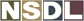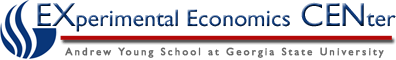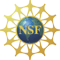Students / Subjects# Derived Properties of Preferences

There are two additional relations, called indifference and strict preference that can be defined in terms of the preference relation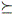. Both of these relations inherit the properties of. In order to prove the existence of a utility function that represents choice, transitivity of the strict preference relation is needed.

### Indifference

Two consumption levels x and x' are indifferent if xx' and if x'x. This is typically written x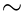x'.

Indifference is transitive because the relationthat it is derived from is transitive.

Proof: If xx' and x'x'', then from the definition of indifference above, xx' and x'x'' so that xx'', and also x''x' and x'x so that x''x. Taken together, this shows that if xx' and x'x'' then xx''.

### Strict Preference

Consumption level x is strictly preferred to consumption level x' if xx' and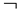x'x. (The symbolmean "not".) In words, x is at least as good as x', but x' is not at least as good as x. This leaves only the possibility that x is strictly preferred to x', which we write as x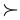x'.

Strict preference inherits transitivity from.

Proof: Let x and x' be such that xx' and x'x''. Then the strict preference relationis transitive if this implies that xx''.

From the definition of strict preference, xx' if and only if xx' and(x'x). Similarly, x'x'' if and only if x'x'' and(x''x'). From xx' and x'x'' and transitivity ofwe get xx''. Suppose that x''x. Since xx' this would imply, again by transitivity of, that x''x'. But x'x'' which by definition implies that(x''x'). This contradicts the assumption that x''x, so xx'' and(x''x), which is equivalent to xx''.

Next